Next: MINIMUM K-VERTEX CONNECTED SUBGRAPH Up: Cuts and Connectivity Previous: MINIMUM B-VERTEX SEPARATOR   Index

### MINIMUM QUOTIENT CUT

• INSTANCE: Graph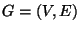, a vertex-weight function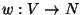, and an edge-cost function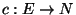.

• SOLUTION: A cut C, i.e., a subset.

• MEASURE: The quotient of the cut, i.e.,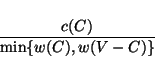where c(C) denotes the sum of the costs of the edges (u,v) such that eitherand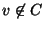or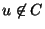and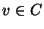and, for any subset, w(V') denotes the sum of the weights of the vertices in V'.

• Good News: Approximable within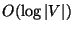.

• Comment: Also called Minimum Flux Cut. Admits a PTAS for planar graphs . The generalization to hypergraphs, also called Minimum Net Expansion, is approximable within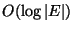in the uniform vertex-weight case . Other approximation algorithms for hypergraph partitioning problems are contained in .

Viggo Kann
2000-03-20